Next: Worked example 5.1: Bucket Up: Conservation of energy Previous: Motion in a general

## Power

Suppose that an object moves in a general force-field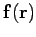. We now know how to calculate how much energy flows from the force-field to the object as it moves along a given path between two points. Let us now consider the rate at which this energy flows. If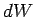is the amount of work that the force-field performs on the mass in a time interval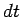then the rate of working is given by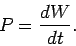(176)

In other words, the rate of working--which is usually referred to as the power--is simply the time derivative of the work performed. Incidentally, the mks unit of power is called the watt (symbol W). In fact, 1 watt equals 1 kilogram meter-squared per second-cubed, or 1 joule per second.

Suppose that the object displaces by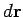in the time interval. By definition, the amount of work done on the object during this time interval is given by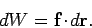(177)

It follows from Eq. (176) that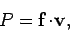(178)

where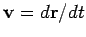is the object's instantaneous velocity. Note that power can be positive or negative, depending on the relative directions of the vectors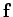and. If these two vectors are mutually perpendicular then the power is zero. For the case of 1-dimensional motion, the above expression reduces to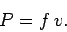(179)

In other words, in 1-dimension, power simply equals force times velocity.Next: Worked example 5.1: Bucket Up: Conservation of energy Previous: Motion in a general
Richard Fitzpatrick 2006-02-02